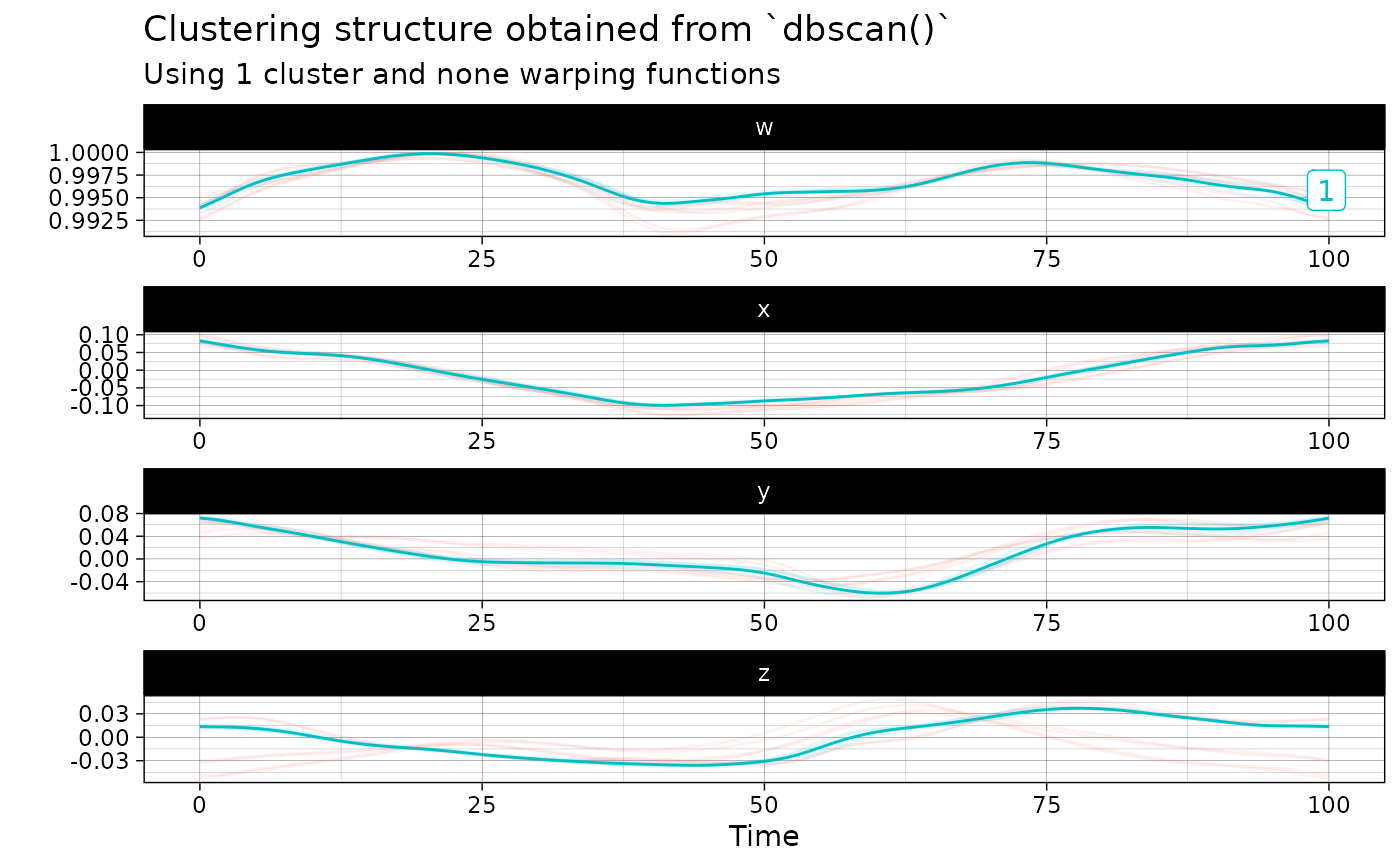This function massages the input quaternion time series to apply DBSCAN clustering on them, with the possibility of separating amplitude and phase variability and of choosing the source of variability through which clusters should be searched.

## Usage

dbscan(x, ...)

# S3 method for default
dbscan(x, eps, minPts = 5, weights = NULL, borderPoints = TRUE, ...)

# S3 method for qts_sample
dbscan(
x,
warping_class = c("affine", "dilation", "none", "shift", "srsf"),
centroid_type = "mean",
metric = c("l2", "pearson"),
cluster_on_phase = FALSE,
use_fence = FALSE,
...
)

## Arguments

x

Either a numeric matrix of data, or an object that can be coerced to such a matrix (such as a numeric vector or a data frame with all numeric columns) or an object of class qts_sample.

...

additional arguments are passed on to the fixed-radius nearest neighbor search algorithm. See frNN() for details on how to control the search strategy.

eps

size (radius) of the epsilon neighborhood. Can be omitted if x is a frNN object.

minPts

number of minimum points required in the eps neighborhood for core points (including the point itself).

weights

numeric; weights for the data points. Only needed to perform weighted clustering.

borderPoints

logical; should border points be assigned to clusters. The default is TRUE for regular DBSCAN. If FALSE then border points are considered noise (see DBSCAN* in Campello et al, 2013).

warping_class

A string specifying the warping class Choices are "affine", "dilation", "none", "shift" or "srsf". Defaults to "affine". The SRSF class is the only class which is boundary-preserving.

centroid_type

A string specifying the type of centroid to compute. Choices are "mean", "median" "medoid", "lowess" or "poly". Defaults to "mean". If LOWESS appproximation is chosen, the user can append an integer between 0 and 100 as in "lowess20". This number will be used as the smoother span. This gives the proportion of points in the plot which influence the smooth at each value. Larger values give more smoothness. The default value is 10%. If polynomial approximation is chosen, the user can append an positive integer as in "poly3". This number will be used as the degree of the polynomial model. The default value is 4L.

metric

A character string specifying the distance measure to be used. This must be one of "euclidean", "maximum", "manhattan", "canberra", "binary" or "minkowski" if x is not a QTS sample. Otherwise, it must be one of "l2", "pearson" or "dtw".

cluster_on_phase

A boolean specifying whether clustering should be based on phase variation or amplitude variation. Defaults to FALSE which implies amplitude variation.

use_fence

A boolean specifying whether the fence algorithm should be used to robustify the algorithm against outliers. Defaults to FALSE. This is used only when warping_class != "srsf".

## Value

An object of class stats::kmeans or stats::hclust or dbscan_fast if the input x is NOT of class qts_sample. Otherwise, an object of class qtsclust which is effectively a list with four components:

• qts_aligned: An object of class qts_sample storing the sample of aligned QTS;

• qts_centers: A list of objects of class qts representing the centers of the clusters;

• best_clustering: An object of class fdacluster::caps storing the results of the best k-mean alignment result among all initialization that were tried.

• call_name: A string storing the name of the function that was used to produce the clustering structure;

• call_args: A list containing the exact arguments that were passed to the function call_name that produced this output.

## Examples

out <- dbscan(vespa64\$igp[1:10])
plot(out)